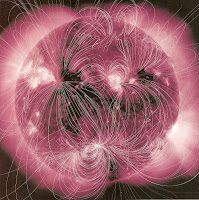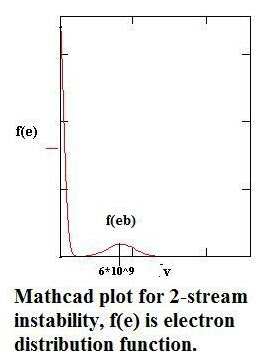## Friday, August 18, 2017

### What Aspects Of The Coming Total Solar Eclipse Are Most Critical For Astrophysics?Image showing totality in a previous  solar eclipse. The Sun is totally blocked out by the Moon - in the line of sight- and the solar corona is seen expanding from the solar limbGraphic showing the path for Aug. 21 total eclipse - and the partial zones to either side, with the percentages of the Sun to be covered.

As we approach August 21 and the first total eclipse to appear in in the U.S. in38 years, many are trying to stay ahead of the fervid hype. Here are the only two things you need to know: 1) In most of the U.S. the eclipse will be seen as partial only and you need special glasses in order to observe the transition to maximum coverup, and 2) If you want to observe the total eclipse (see top image) you will have to travel to some place within the band of totality (see graphic).  For example, in our location (Colorado Springs) the Sun will be roughly 85 percent covered.

Here's the skinny on stats for this eclipse: Approximately 12 million Americans will be directly in the totality band shown in the graphic. Another 88 million will be within 200 miles of some place inside the totality band. Naturally then, those living in communities right in the band (e.g. Jackson Hole, WY, St. Joseph, MO) are expecting eclipse watchers to pour in and pump up their economies - buying t-shirts, special glasses and other paraphernalia.

While the basic image of totality will be the appeal for casual observers,  and even partial eclipse will occur for (and excite)  many others, this doesn't hold quite the same spell for solar physicists or astrophysicists. They look to good observations made during totality, namely to be able to record and analyze the solar corona.   This is the outermost region of the solar atmosphere, at millions of kelvins temperature and extending sometimes millions of kilometers into space.

The corona up to now has presented a mystery, especially in terms of its "inverse" increasing temperature profile considered in the context the general temperature profile is steadily decreasing from the Sun's interior to outside.   For example, the Sun's "surface" or photosphere has an effective temperature of 5,777 K .   The corona's temperature by contrast is at least 2 million degrees K.

How did we find this out?  The key breakthrough probably arrived in 1939 when astronomer Walter Grotrian found that a previously discovered spectral line (attributed to "coronium") was actually 13 times ionized iron. Since it takes enormous energy to ionize even one iron atom (meaning stripping one of its outer electrons away) this meant the iron had to be subject to enormously high temperatures.  Indeed, such ions can only exist in plasmas with temperatures between 1 million and 5 million K.

The first clue as the cause of the extraordinary hearing came with high altitude rocket flights in the 1970s, bearing x-ray telescopes. These showed  features down to a resolution of 1 arcsec or 730 km.  Careful observations showed a positive correlation between the x-ray brightness of active regions (i.e. containing complex sunspots) and plasma-filled magnetic loops, e.g.The above image was taken by the much more recent Atmospheric Imaging Assembly, built for the Solar Dynamics Observatory . But you easily get the idea noting the brightness (representative image taken at  211Å) and the magnetic loop structures.

Another milestone occurred when Eugene Parker predicted the hot corona must expand into space as a solar "wind". It could not remain stationary or in place with no expansion. The proof of this is left at the end for those interested.

The next question, of course, is where the energy comes from not only to heat the corona but accelerate the solar wind which is an extension of it.  We believe now that MHD or "magnetohydrodynamic" waves of some type provide an answer.   Field lines such as shown in the previous image are rooted at both ends in the Sun's convection zone where they are jostled by the churning of convective cells.  If sufficiently rapid these field line motions could generate magnetic waves capable of carrying energy upward into the loop.   It seems that Alfven waves e.g.

http://brane-space.blogspot.com/2011/04/getting-handle-on-alfven-waves-2.html

are the most effective in reaching coronal heights. (Alas, Alfven waves do not compress the ambient plasma so we still need to identify some other mechanism that can effectively transfer the wave energy to the plasma. A clue may lie with Landau damping, say associated with a beam (or 2-stream)  instability in the plasma. We have a dual Maxwellian profile.In the region where the slope is positive (f(v)  / v > 0) there is a greater number of faster than slower particles so a greater amount of energy is transferred from particles to associated (e.g. Alfven) waves.  Since f eb contains more fast than slow particles a wave is excited.

But in Landau damping, with the slope negative (f(v)  / v < 0) the number of particles slower than the waves phase velocity exceeds the number of those that are faster. Thus, more particles gain energy from the wave than lose energy to it.

Whatever new data the eclipse generates, and there will be lots of it, we can be confident that the mysteries of the solar corona won't be solved even after it's all analyzed and multiple papers published. But I will keep readers abreast of any new findings say that appear in the next 8-12 months.

Those interested in actual scientific participation are invited to go to the Globe Observer site, where you can collect the app and then be prepared to make temperature measurements.

https://observer.globe.gov/

----------
Proof that the corona can't be stationary:

A static corona  superficially appears  to be quite reasonable but that’s why we need to test this is so. The first one to do this was Sydney Chapman. He began by first assuming the condition for hydrostatic equilibrium applied:

dp/ dr = -
r {GMs/ r2}

where G is the usual Newtonian gravitational constant, and
r defines the plasma density for the corona, while Ms is the mass of the Sun, and r the distance from the solar center:

r = n(mp)

with n the number density for protons

The coronal pressure (P) is given by:

P = 2 n T

Provided both protons and electrons are assumed to have the same temperature.

The thermal conductivity of the corona is dominated by electron thermal conductivity and takes the form:

k = ko T 5/2

for typical coronal conditions the value of
k is about 20 times the value of copper at room temperature.

The coronal heat flux density is:

q = -
k Ñ T

A static corona means heat inputs cancel heat outputs so that the divergence:

Ñ× q = 0

Assuming a spherical symmetry for the corona one can write:

1/r2 [d/dr (r2
ko T 5/2  dT/dr)] = 0

Obviously the preceding assumptions mean there must be some distance where the coronal temperature becomes zero.

From the above equation one should be able to show:

d(T 7/2) = 7/2 (F To 5/2)/ 4
p ko d(1/r) = C d(1/r)

where C is a constant.

The integral is:

To 7/2 - T 7/2 = C[ 1/Ro - 1/r]

Now, set the temperature at infinity (T) to zero and obtain:

C = Ro To 7/2

which fixes the total flux at:

F = 2/7   [4
p Ro ko To ]

After another step, one finds:

T(r) = To (Ro / r) 2/7

This gives the temperature T at a distance from the Sun= r. This is based on using a defined value (say To = 2 x 106 K) at a defined distance, say Ro = 7 x 108 m.

For example, at the Earth’s distance (r = 1.5 x 1011 m) one would find: T = 4.3 x 105 K

This seems fine, until one examines the pressure.

Analogous to the temperature formalism, we have, the pressure p(r) at some distance r defined by:

p(r) =

p(Ro) exp [7/5 GMs mp/ 2 T(Ro) Ro {( Ro / r)5/7 – 1}]

Now, if one allows r to approach infinity, e.g. r
®¥ an interesting thing occurs in the equation, as we can see. That is, the denominator of the first term in the end brackets becomes so large (Ro / ¥) that the first term vanishes.

Then we are left with the expression for the pressure:

p(
¥) = p(Ro) [exp – 7k/5 * 1/ T(Ro) Ro]

where ‘k’ denotes a constant composed of all the constant quantities in the previous eqn. (G, M, mp etc)

Substituting the given values into the above, one finds p(Ro) multiplied by a factor

exp = 1

The reason is that the exponential of a very small and negative valued magnitude
® 0

Then:

p(
¥)  »  p(Ro)

But this can’t be since the pressure of the coronal base would then be the same as the value at infinity!

This led astrophysicists to conclude an unphysical result, and that the static coronal model couldn’t be accurate.

If the static model were accurate, the pressure at infinity should be zero, p(
¥)   = 0, not a small finite pressure that’s effectively equal to the coronal base pressure. This finding led to the further investigations that disclosed a solar “wind” had to flow outwards from the corona.